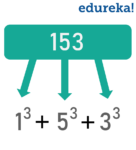# How to check if a given number is an Armstrong number or not?2 / 29 Blog from Java Programs

In number theory, a narcissistic number, an Armstrong number is named after Michael F. Armstrong is a number that is the sum of its own digits each raised to the power of the number of digits. In this Armstrong Number in Java article, let’s learn how to check whether a given number is Armstrong number or not.

Let’s begin!

## What is an Armstrong Number?

The sum of the power of individual digits is equal to the number itself. Between 1 to 1000, there are five Armstrong numbers. They Are :- 1, 153, 370, 371, 407. Here’s the general equation.

```abcd... = an + bn + cn + dn + ...```

Let’s check out the concept with some examples.
Example1: 370

3*3*3 + 7*7*7 + 0*0*0 = 27 + 343 + 0 = 370

Example2: 407
4*4*4 + 0*0*0 + 7*7*7 = 64 + 0 + 343 = 407

I hope that you are clear with the concept now. Moving on, let check out how to check whether a given number is Armstrong number or not in Java.

## Java Program to check an Armstrong number

You can check whether a given number is Armstrong number or not in Java in two ways:

1. Using ‘while’ loop
2. Java ‘for’ loop

### Using ‘while’ loop

In case of an Armstrong number of 3 digits, the sum of cubes of each digit is equal to the number itself. The example program below checks if a given 3 digit number is Armstrong number or not.

```package MyPackage;

public class ArmstrongNumber{
public static void main(String[] args) {
int num = 371, originalNum, remainder, result = 0;
originalNum = num;
while (originalNum != 0)
{
remainder = originalNum % 10;
result += Math.pow(remainder, 3);
originalNum /= 10;
}
if(result == num)
System.out.println(num + " is an Armstrong number.");
else
System.out.println(num + " is not an Armstrong number.");
}
}

```

Output: 371 is an Armstrong number.

The steps listed in the code are:

• The first line in while loop extracts the last digit (remainder) from the number specified
• The second line calculates the cube of the last digit taken from the previous step and adds it to the result
• Then, the last digit is removed from originalNum after division by 10

### Using ‘for‘ loop

```package MyPackage;

public class Armstrong {
public static void main(String[] args) {
int number = 9474, originalNumber, remainder, result = 0, n = 0;
originalNumber = number;
for (;originalNumber != 0; originalNumber /= 10)
{
n++;
}
originalNumber = number;
for (;originalNumber != 0; originalNumber /= 10)
{
remainder = originalNumber % 10;
result += Math.pow(remainder, n);
}
if(result == number)
System.out.println(number + " is an Armstrong number.");
else
System.out.println(number + " is not an Armstrong number.");
}
}```

Output:

```9474 is an Armstrong number.
```

Here, we have two for loops. The first one calculates the number of digits in the given number. The second loop checks if the given number is Armstrong number or not.

Check out the Java Certification Course by Edureka, a trusted online learning company with a network of more than 250,000 satisfied learners spread across the globe. We are here to help you with every step on your journey, for becoming a besides this java interview questions, we come up with a curriculum which is designed for students and professionals who want to be a Java Developer.

Got a question for us? Please mention it in the comments section of this “Armstrong number in Java” blog and we will get back to you as soon as possible.

Upcoming Batches For Java Certification Training Course
Course NameDate
Java Certification Training Course

Class Starts on 25th February,2023

25th February

SAT&SUN (Weekend Batch)
View Details
Java Certification Training Course

Class Starts on 25th March,2023

25th March

SAT&SUN (Weekend Batch)
View DetailsREGISTER FOR FREE WEBINARThank you for registering Join Edureka Meetup community for 100+ Free Webinars each month

### Subscribe to our Newsletter, and get personalized recommendations.#### How to check if a given number is an Armstrong number or not?

edureka.co